GFG App
Open AppBrowser
Continue

Wheatstone bridge is used To find the resistance of a conductor, in 1842, scientist Wheatstone proposed a theory, which is called the principle of Wheatstone bridge after his name. we can prove or establish the formula for Wheatstone by using Kirchhoff laws. Wheatstone bridge is simply an electric circuit used to measure an unknown electric resistance by balancing two-point of a bridge. Let’s get started!

### What is Kirchhoff’s law?

In 1842, the scientist Kirchhoff formulated two laws to find the distribution of current in different conductors in any complex electric circuit. These rules are as follows:

• First law: The algebraic sum of all the electric currents found at each junction of an electric circuit is zero. In mathematical terms, on any junction: ΣI = 0. The currents coming towards the junction are taken as positive and the currents going away from the junction are taken as negative. In other words, when a constant current flows in a circuit, neither charge is accumulated nor removed from any junction in the circuit, that is, Kirchhoff’s law is based on the principle of conservation of charge. It is also called Kirchhoff’s law of current.
• Second law: In any closed electric circuit, the algebraic sum of the products of the currents flowing in different sections and their corresponding resistances (i.e. potential difference) and the different electro-carrying forces applied in that circuit is zero. In mathematical language, ΣIR + ΣE = 0. Kirchhoff’s second law is actually the law of conservation of energy, according to which there should be no change in its energy when a charge moves in a completely closed circuit. It is also called Kirchhoff’s voltage law.

Principle of Wheatstone Bridge:

According to this principle, four resistors P, Q, R and S are placed in the form of four sides AB, BC, AD and DC of a quadrilateral ABCD. A cell E and key K1, is placed between the A and C ends of this quadrilateral and a sensitive galvanometer G and key K2, is placed between the B and D ends. Clearly, the potential of point A will be equal to the potential of the positive plate of the cell and the potential of point C will be equal to the potential of the negative plate of the cell.

It is clear from the figure that the resistances P and Q are in series when the key K2 is open. Similarly, resistances R and S are in series, but P and Q together (arm ABC) and R and S together (arm ADC) are connected in parallel to each other. Since the side BD of the galvanometer is placed like a bridge over the sides ABC and ADC of the quadrilateral, this circuit is called a bridge.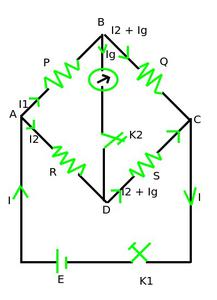When the bridge is in an equilibrium state, that is, there is no deflection in the galvanometer. That is, in the equilibrium state of the bridge, the ratio of the resistances of any two adjacent arms is equal to the ratio of the remaining two adjacent sides.Establishment of formulas from Kirchhoff’s law:

Suppose, on pressing the cell key K1, a current I flows through the cell, which splits into two parts at the end A. One part I1 flows through the resistance P in the arm AB and the other part I2, through the resistance R in the arm AD. The current I1 again comes to end B and gets divided into two parts. One part of it Ig flows through the galvanometer in arm BD and the remaining part (I1– Ig) flows through resistance Q in arm BC. At the end D, the current I2 from arm AD and the current Ig from arm BD, so the current flowing through the resistance S in arm DC will be (I2 + Ig).

So according to Kirchhoff’s law, in closed path ABDA,

I1P + IgG – I2R = 0                                                                                                                                                                            ……(1)

And in closed path BCDB,

(I1 – Ig)Q – (I2 – Ig)S – IgG = 0                                                                                                                                                             ……(2)

The values ​​of the resistors P, Q, R and S are taken in such a way that no current flows through the galvanometer G when the key K2, is pressed. This is called the equilibrium state of the bridge, that is, in the equilibrium state of the bridge, the deflection in the galvanometer is zero (Ig = 0).

Putting Ig = 0, in above equations,

I1P = I2R and I1Q = I2S

or

I1P / I1Q = I2R / I2S

or

P / Q = R / S

This is the necessary condition for the balance of the Wheatstone bridge. With the help of the above formula, knowing the values ​​of three resistors P, Q and R, the value of the fourth resistance S can be found.

Generally, the arms with resistance P and Q are called ratio arms. The arm AD with resistance R is called the known arm and the arm CD with resistance S is called the unknown arm. In the equilibrium position of the bridge, changing the position of the cell and the galvanometer does not affect the state of equilibrium (in this case the potential of points A and C will be the same), so the arms AC and BD are conjugate arms. are called. The sensitivity of the bridge is highest when the resistances of all four arms are almost equal.

It is to be remembered that in the equilibrium state (when P / Q = R / S), the total resistance of the circuit between points A and C will be equal to (P + Q) × (R + S) / (P + Q) + (R + S) [because the resistance of arm ABC is (P + Q) and resistance of arm ADC (R + S) are connected in parallel order] and the potentials of points B and D will be same.

It is clear that if the potential of point B is more than the potential of point D, then by pressing the galvanometer key K2, the current will start flowing from point B to D, which will deflect the galvanometer to one side. Conversely, if the potential at point D is greater than the potential at point B, the current will flow from point D to B as the galvanometer key K2 is pressed, causing the galvanometer to deflect in the opposite direction.

• With the help of Wheatstone’s Bridge, we can build a Meter bridge.
• The biggest advantage of Wheatstone’s Bridge is to accurately measure the electric resistance instead of using costly instruments.
• We can measure minute changes in the bridge, even in m ohm.
• It is very easy to find out the unknown resistance as the rest of the three are easily known.
• We can measure strain and pressure using a Wheatstone bridge.

• The result of Wheatstone’s Bridge can be easily affected by temperature and EMF cells.
• The Wheatstone bridge may also get affected if the galvanometer is not of good quality.
• The Wheatstone bridge fails if it is not in a balanced condition.
• We can’t measure large resistance with the help of Wheatstone’s Bridge.
• The cost of maintaining the Wheatstone’s Bridge is very high.

### Meter Bridge

The meter bridge is shown in the figure. It is made of a one-meter long wire of uniform cross-sectional area which is clamped vertically between two thick plates of metal, as shown. There are two gaps in the metallic plate between which resistors can be connected. The endpoints where the wires are held are connected to the cell by a key. One end of the galvanometer is connected to a metallic plate in the middle of the gap. The other end of the galvanometer is connected to the jockey. A jockey is essentially a metallic rod with a sharp edge at one end and which can be glide over a wire to form an electrical connection. R is an unknown resistance whose value we wish to find. Combine it in either of the spaces.  A standard known resistance S is connected between the second spacing.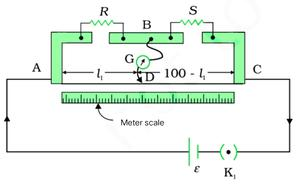Touch a point D on the jockey’s wire which is at a distance of l cm from end A. You can slide the jockey along the wire. The resistance of the AD part of the wire is Rcm l where Rcm is the resistance per unit cm of the wire. Thus the resistance of the DC part is R cm ( 100 – l ).

Four arms AB, BC, DA and CD [whose resistances are R, S, Rcm l and Rcm ( 100 – l ) respectively ] obviously form a Wheatstone bridge with AC battery arm and BD galvanometer arm. If the jockey is moved along the wire, there will come a point (equilibrium point) where the galvanometer will not show any current. Let the distance of the jockey at the equilibrium point be = 1, then the values ​​of the four resistors of the bridge at the equilibrium point are R, S, Rcm l1 and Rcm (100 – l1). According to Equation Wheatstone’s bridge for the equilibrium condition.

R / S = Rcm l1/ (Rcm (100 – l1

= l1 / 100 – l1

As soon as we find the value of l1, the  unknown resistance R is obtained in terms of the standard known resistance S. therefore:

R = S ( l1 / 100 – l1)

By choosing different values ​​of S, we get different values ​​of l1 and calculate R each time. Of course, an error in the measurement of l1 will result in an error in R. It can be shown that the percentage error in R can be minimized by adjusting the equilibrium point by placing  l1 closer to the middle of the bridge wire, 50 cm (this requires a proper choice of S).

### Sample Questions

Question 1: Find the equivalent resistance between points A and C in the circuit shown in the figure below: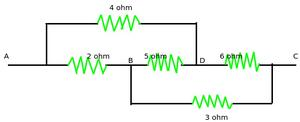The equivalent circuit of the circuit shown in the above figure is given as: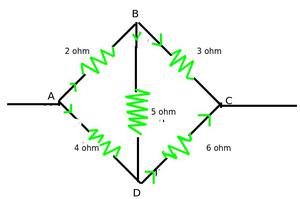Since,

2/3 = 4/6, this is the circuit of a balanced Wheatstone bridge.

In the balanced state, VB = VD, so no current will flow through the 5 Ω resistance.

Now the equivalent resistance of sides AB and BC is R’ = 2 + 3 = 5 Ω.

The equivalent resistance of AD and DC arm R” = 4 + 6 = 10 Ω

If the equivalent resistance between the points A and C is R, then.

1 / R = (1 / R’) + (1 / R”)

or

1 / R = (1 / 5) + (1 / 10)

=   1 / R = (2 + 1) / 10

or

R = 10 / 3

= 3.33 Ω

Question 3: The electric circuit of a balanced Wheatstone bridge is shown in Figure. Calculate the resistance x.

Let the total resistance in arm BC be R. Since the bridge is balanced, therefore: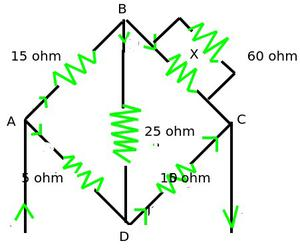15 / R = 5 / 10

R = (15 × 10) / 5 = 3 Ω

1 / R = (1 / X) + (1 / 60)

or

1 / 30 = (1 / X) + (1 / 60)

or

1 / X = 1 / 30 – 1 / 60

1 / X = 2 – 1 / 60 = 1 / 60

or

X = 60 Ω

Question 4: What is Meter Bridge? And what kind of precautions do we need to perform measurement using Meter Bridge?

A Meter bridge is a device based on the principle of the Wheatstone bridge, with the help of which the resistance and specific resistance of a conductor can be determined. In this, a 1-meter long wire acts as the proportional side.

Precautions while using Meter Bridge: Precautions are:

1. The ends of all the connection wires should be cleaned with sandpaper.
2. The current should not flow in the circuit for a long time otherwise, its resistance increases due to the heating of the bridge wire. Therefore, the key in the cell circuit should be plugged in only when observations are to be made.
3. The jockey should not be run by rubbing it on the meter bridge wire otherwise, the thickness of the wire will not remain the same at all places.
4. A shunt with a galvanometer should be used initially while adjusting, but the shunt should be removed near the position of zero deflection.
5. Only such a resistance plug should be removed from the resistance box so that the position of zero deflection is approximately in the middle of the bridge wire. In this case, the sensitivity of the bridge is maximum and the percentage error is minimum.
6. All other plugs in the resistance box, except those that have been removed, should be tightly packed.

Question 5: In the following figure the current through 4Ω resistor is?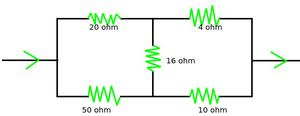Since, Q / P = S / R

⇒ 4 / 20 =10 / 50

It is an example of balanced wheat-stone bridge.

So, No current will flow through 16 Ω resistance.

As we know that current divide in inverse ratio, current through 4Ω resistance is,

= 1.4 × (20+4)+(50+10) / (50+10)

= 1.4 × (7/5)

= 1 A

My Personal Notes arrow_drop_up